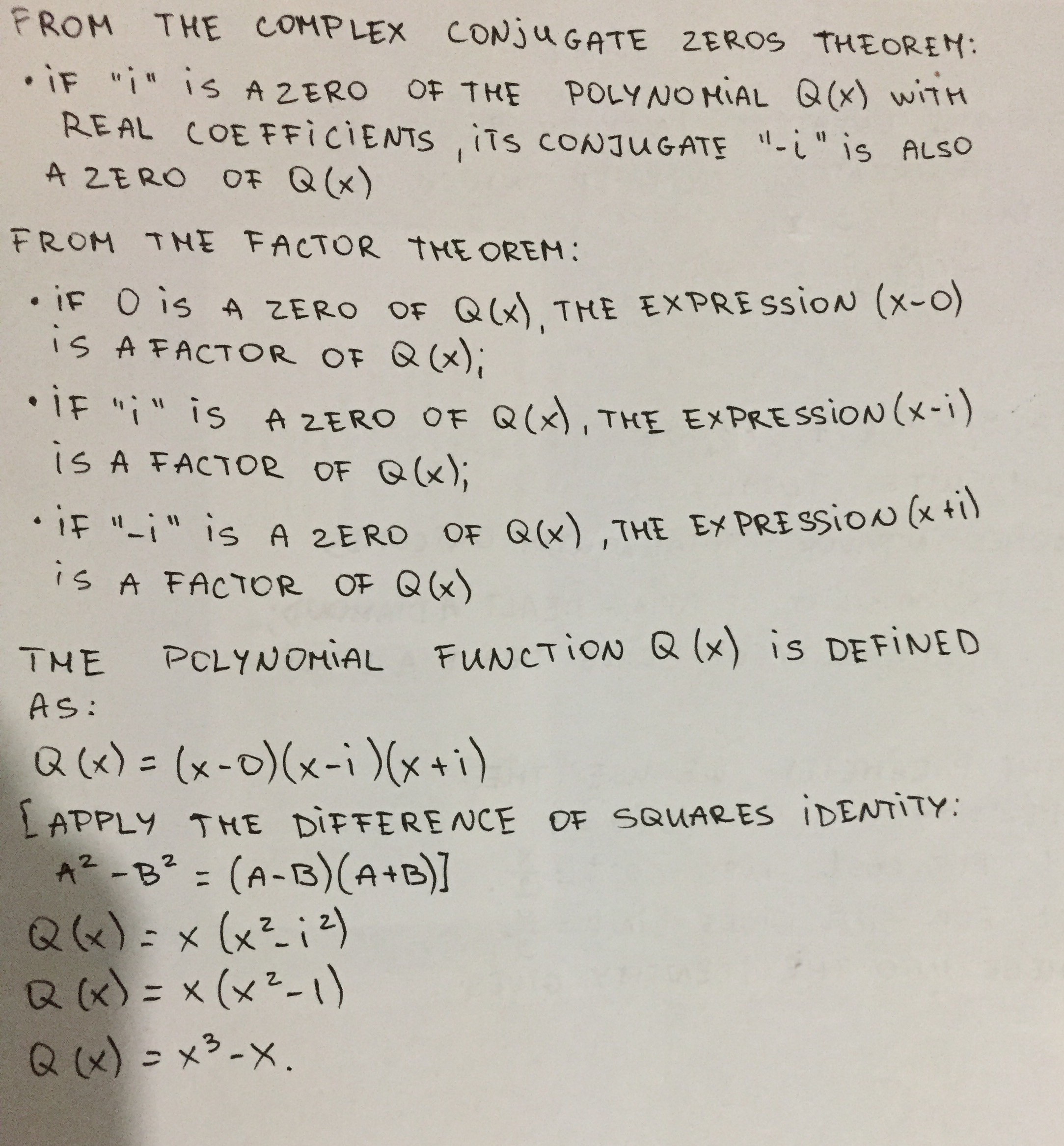# Find a polynomial with integer coefficients that satisfies the given conditions. Q has degree 3 and zeros 0 and i.Albarellak 2020-10-28 Answered
Find a polynomial with integer coefficients that satisfies the given conditions. Q has degree 3 and zeros 0 and i.
You can still ask an expert for help

• Questions are typically answered in as fast as 30 minutes

Solve your problem for the price of one coffee

• Math expert for every subject
• Pay only if we can solve itLiyana Mansell

From the Complex Conjugate Zeros Theorem:
If “i” is a zero of the polynomial Q(x) with real coefficients, its conjugate “-i” is also a zero of Q (x)
From the factor theorem:
If 0 is a zero of Q (x) the expression (x – 0) is a factor of Q (x).
If “i” is a zero of Q (x) the expression (x – i) is a factor of Q (x).
If “-i” is a zero of Q (x) the expression (x + i) is a factor of Q (x).
The polynomial function Q (x) is defined as:
$$\displaystyle{Q}{\left({x}\right)}={\left({x}–{0}\right)}{\left({x}–{i}\right)}{\left({x}+{i}\right)}$$
[apply the difference of squares identity: $$\displaystyle{a}^{{2}}–{b}^{{2}}={\left({a}-{b}\right)}{\left({a}+{b}\right)}{]}$$
$$\displaystyle{Q}{\left({x}\right)}={x}{\left({x}^{{2}}–{i}^{{2}}\right)}$$
$$\displaystyle{Q}{\left({x}\right)}={x}{\left({x}^{{2}}+{1}\right)}$$
$$\displaystyle{Q}{\left({x}\right)}={x}^{{3}}+{x}$$